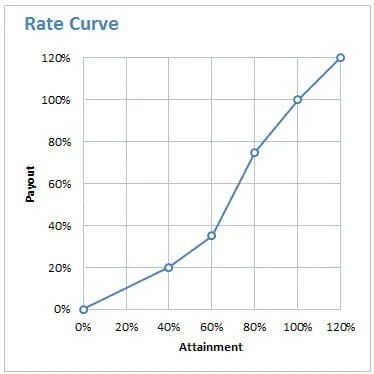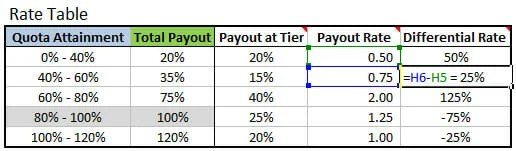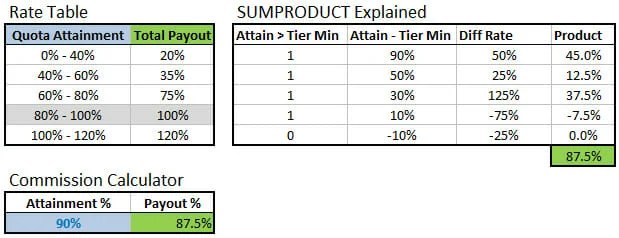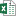# Excel Formula to Calculate Commissions with Tiered Rate Structure

Excel Formula to Calculate Commissions with Tiered Rate Structure

In this post I will explain how to calculate a dollar or percentage commission payout in one cell using the SUMPRODUCT function.  Calculating commissions on a tiered rate structure can be difficult because you are trying to determine the cumulative payout based on different rates at each tier, and the achievement amount might fall in between one of the tier ranges.

If your commission plan tiers are not cumulative, then you might want to checkout my article on how to calculate commissions with VLOOKUP.  This is a simpler calculation then the one presented below.

### Tiered Rate Table

The following is an example of a tiered rate table for sales commissions.  The first column contains the tiered ranges of Quota Attainment and the second column contains the Payout % for each tier.  If the sales person (rep) achieves sales that are at the top end of each tier, then they will receive the full payout amount in the Total Payout column.  For example, if the rep sells 40% of their quota then they will receive 20% of their commission.  If they sell 60% of quota, they will receive 35%, and so on down the table.The difficult part is when the attainment amount falls in between the ranges.  What if the rep sells 50% of his/her quota?  The rep would receive 20% payout for the first 40% of quota, and an additional 7.5% payout for the last 10% of quota.  The last 10% of quota attainment is calculated by finding the payout rate at each tier.  So the total payout on 50% of quota would be 27.5%.

Typically we would have to calculate the payout at every tier and then sum the payout amounts to get the total amount.  Or we could use some complicated IF statement to determine the payout all in one formula.  But there is an easier way…

### The Solution

The SUMPRODUCT function can be used to calculate the entire payout.  First we have to calculate the differential payout rate for each tier.  The differential rate is the difference between the payout rates at each tier.

The payout rate at each tier is the total percentage of payout in the tier, divided by the total percent of attainment possible in the tier.  This is basically the amount paid for each percentage increase in attainment in each tier.  In the image below the Payout Rate for the 0%-40% range is 0.50.  This means that for every 1% attained, the payout will be 0.50 of the 20% total payout.

Payout Rate =([tier attainment max] – [tier attainment min]) / ([payout % this tier] – [payout % previous tier])

### Rate Curve

The payout rate is also known as the rate curve.  The rate curve is displayed below, and you can think of the payout rate as the slope of the curve at each tier.### Differential Rate

The differential rate is calculated by taking the difference between the payout rate in the current tier and the payout rate in the previous tier.

Differential Rate = [payout rate Current tier] – [payout rate Previous tier]This is used in the cumulative calculation of the payout percentage.  As the attainment moves up into multiple tiers, the amount of attainment left in each tier is multiplied by the differential rate.  The sum of all these is the total payout.

### SUMPRODUCT Explained

The SUMPRODUCT formula for Total Payout is:

=SUMPRODUCT( (Attainment > [Tier Min]) * (Attainment –  [Tier Min]) * [Differential Rate] )

Variables in brackets [] refer to entire column in rate table.The following splits the SUMPRODUCT formula into multiple columns and rows for a clearer visual of how the formula is calculating the total payout.

1. Attain > Tier Min: Returns a “1” if the attainment is greater than the attainment tier minimum.  If attainment is 90%, then the condition is true for the first 4 rows and a value of 1 is returned.
2. Attain – Tier Min: Finds the difference between the total attainment and attainment tier minimum. This is necessary because we are multiplying it by the differential rate.  So in the first row we are taking the entire attainment of 90% and multiplying it by the diff rate of 50%.  Each subsequent row is taking the leftover attainment for its tier range, and multiplying it by the payout rate that is leftover for its tier (the diff rate).
3. Diff Rate: The differential rate for each tier.
4. Product: Column 1 * Column 2 * Column 3
5. Sumproduct: Sum of the Product column.  The total payout on 90% attainment is 87.5%### SUMPRODUCT Visualization

The following is a visual example of the Product column plotted on the rate curve.  Sometimes it is easier to understand when you see it visually.  The SUMPRODUCT formula finds the total payout in each tier based on the remaining balance of attainment multiplied by the payout rate in that tier.  This is basically a continuation of the rate curve at each tier to the total attainment of 90%.  In the chart below you can see that the dark grey lines follow the rate curve at each tier and then continue on the same curve to the 90% attainment (green) line.1. For the first tier it is (90%-0%) * (50%-0%) = 45%.  50% is the payout rate for tier 1, and 90% is the total attainment.
2. The second tier is (90%-40%) * (75%-50%)  = 12.5%.  The 90%-40% is the remaining balance of attainment for tier 2, which is 50%.  45% (tier 1) + 12.5% (tier 2) = 57.5%
3. Tier 3 is (90%-60%) * (200%-75%) = 37.5%.  The sum of the first three tiers is 95% (45%+12.5%+37.5%), which is higher than the actual payout of 87.5%.
4. Tier 4 is (90%-80%) * (125%-200%) = -7.5%.  The negative product is a result of the payout rate being less in tier 4 than tier 3.  Or, the slope of the rate curve is flatter in tier 4 than tier 3.  That brings the total payout back down to 87.5%.

### Negative Differential

This negative differential rate in tier 4 is important to note.  Not only does it make for a confusing calculation, it also tells you that the rate of compensation is not as great in tier 4.  For each additional percentage point of attainment, the sales rep is compensated at a lower rate than tier 3.  This might mean that there is more emphasis for the rep to attain sales on their quota in tier 3.  And the monetary motivation is not as great for attainment in tier 4.

Negative differential can also mean that the rate curve is poorly designed.  If the goal is to achieve 100% attainment of quota, then it is probably best to increase the payout rate (rate curve slope) in each tier up to 100%.  This design would give the rep more motivation (higher payout rate) as he/she gets closer to achieving their goal of 100% attainment.

### Apply Formula to a List

Now that we are able to calculate the payout in one cell using one SUMPRODUCT formula, we can apply the formula to a whole list of employees in a table.  See the Total Payout % column  on Sales Table tab of the example file.  This is the major benefit of this formula.  The entire calculation can be handled in one cell and it is easily transferable to other models.  There are no hard-coded variables in your formula, or ugly IF statements.Tiered Commission Rates using SUMPRODUCT.xls (101.4 KB)

Here is a file that uses whole number (units or dollars) for the tiers and payouts, instead of percentages.Commission Plan SUMPRODUCT Units Dollars.xlsx (10.6 KB)

Here is an alternate solution submitted by Matthew Burgos using VLOOKUP instead of SUMPRODUCT.  He explains the formula in detail in the comment below.Tiered Commission Rates Using VLOOKUP.xlsx (12.1 KB)

### Still Confused? 🙂

I was too the first time I learned this technique.  It’s definitely complex.  I’d recommend reviewing it a few times with sample workbook and then try to implement it in your own model.

I have another article on how to calculate commissions with VLOOKUP that is an easier calculation for a simple commission plan.